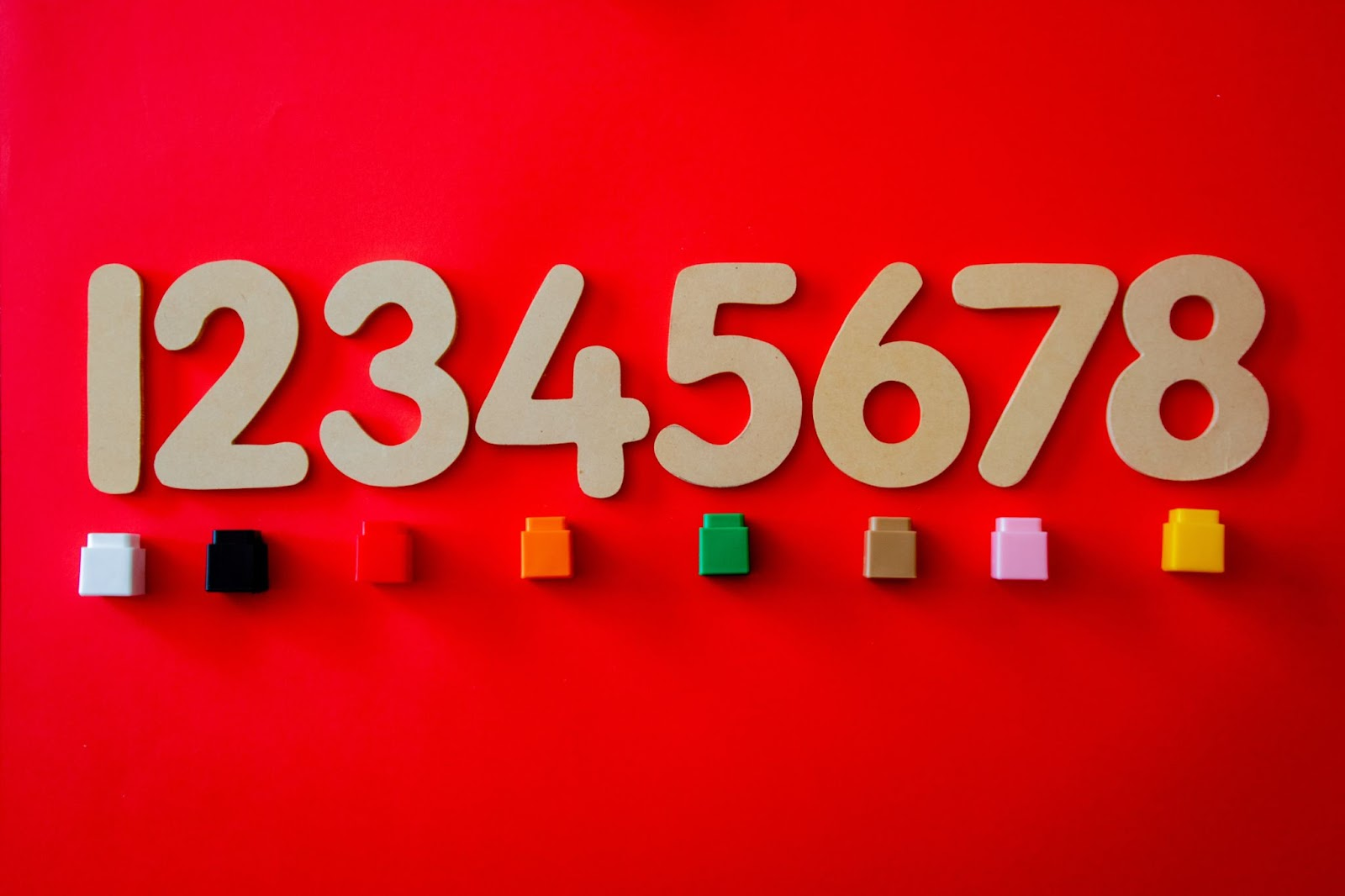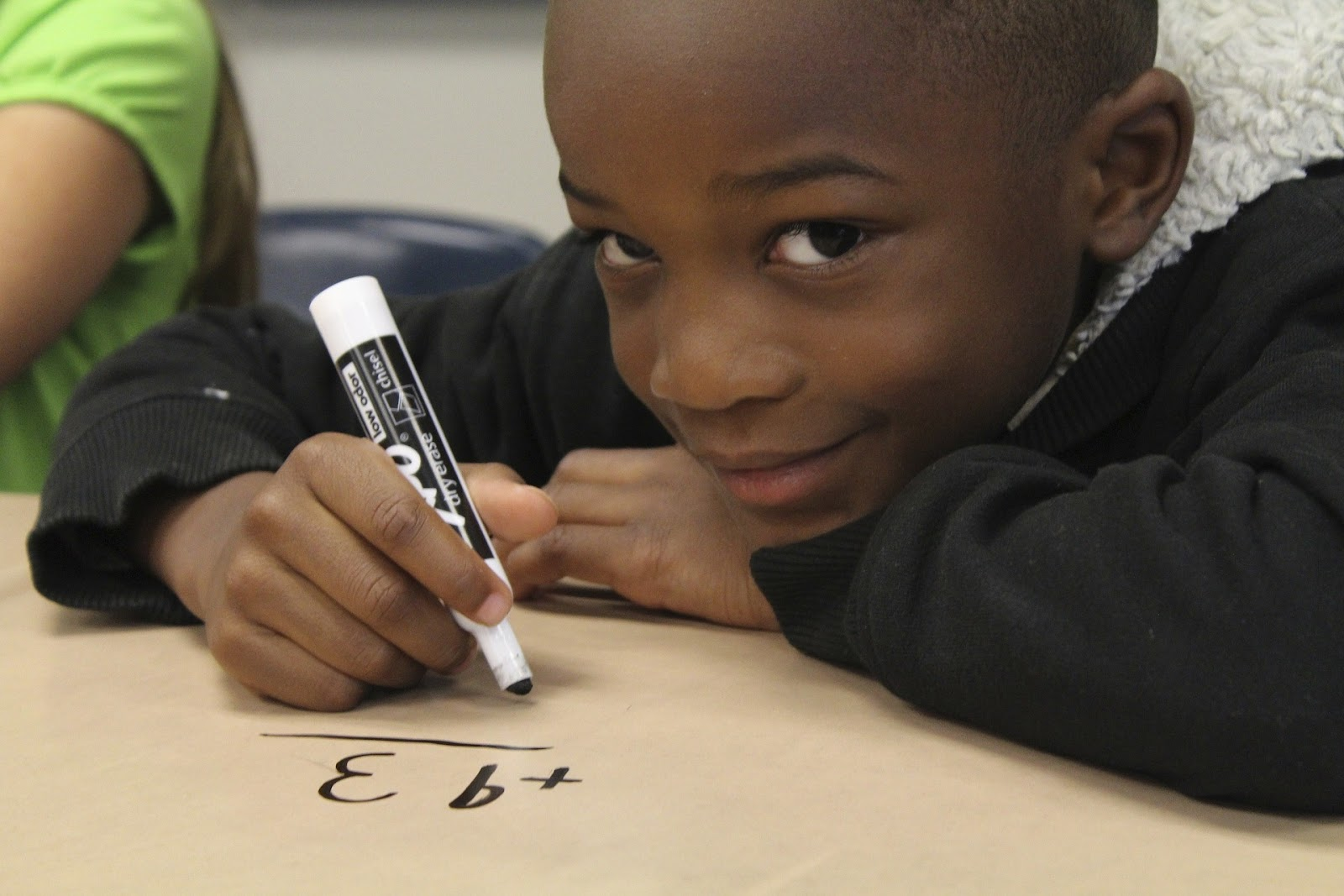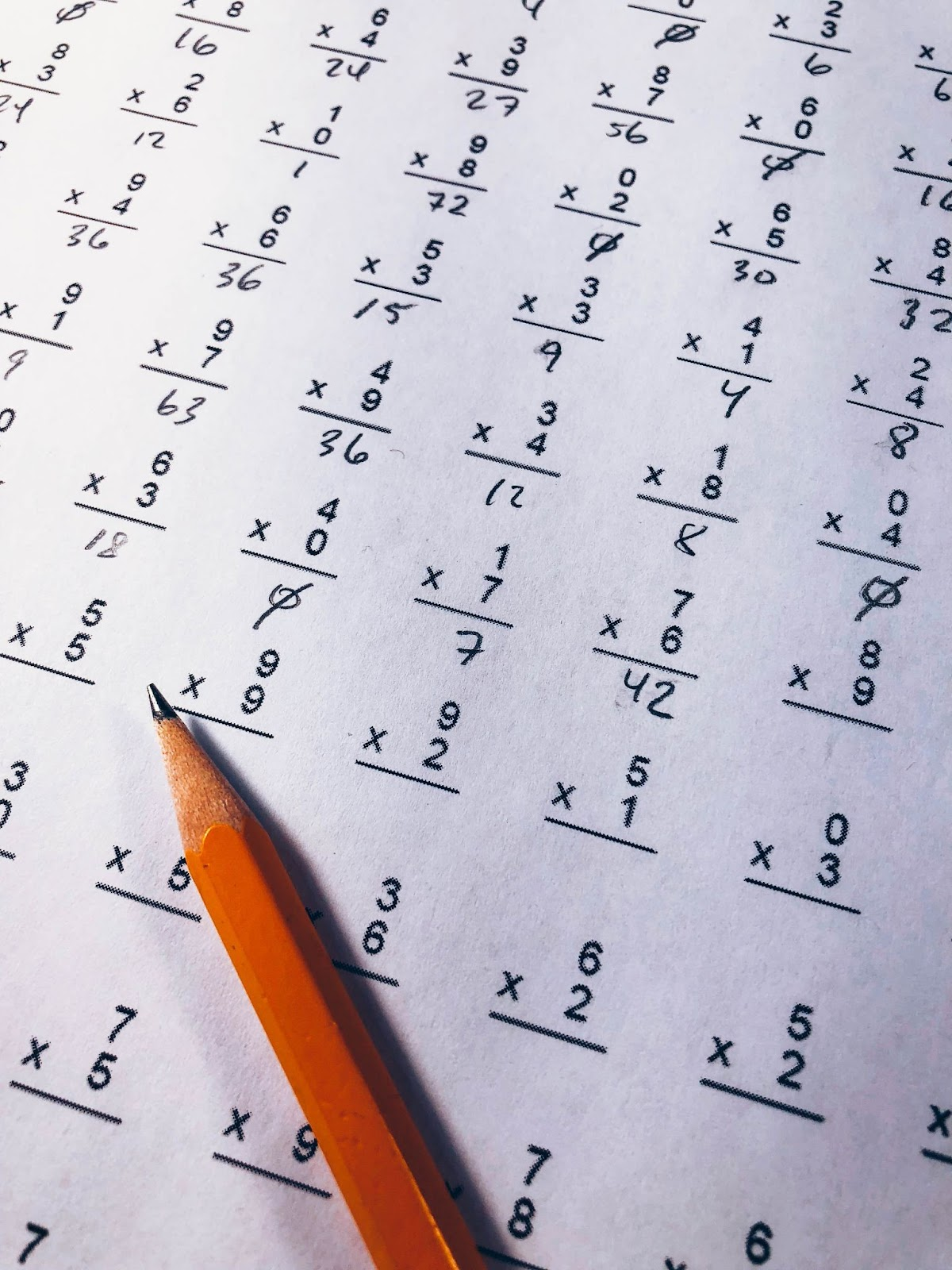## Sunday, July 18, 2021

### Using the CPA Approach to Teach Math

Do your students struggle to retain the concepts that you are teaching? Do you often find yourself asking "Why don't my kids get it?!".

If this sounds like you and your students, this post is exactly what you need to transform your math instruction!

#### What is the CPA Approach?

The CPA Approach was created by psychologist Jerome Bruner and stands for concrete, pictorial, and abstract learning. Jerome Bruner proposed this approach as a means of scaffolding learning. The CPA Approach builds on a child's existing knowledge by introducing abstract concepts in a concrete and tangible way. It’s learning that transitions from concrete materials, to pictorial representations, to abstract symbols and problems.Concrete: Using physical objects to solve math problems. This is a ‘hands-on' approach using real objects and it is the basis for understanding math concepts.

Pictorial: Using drawings to solve math problems. It is sometimes referred to as the “seeing” stage.

Abstract: Solving math problems using only numbers. It is sometimes called the “symbolic” stage.

#### Why should I use the CPA Approach?

Math is abstract and can be confusing for students! That's why providing concrete learning is so important in teaching elementary math. By using concrete materials students are able to ‘see’  the math, and make sense of what is happening. The CPA Approach makes learning math accessible to all students, including those with math learning disabilities.There is a common misconception that older students do not need to use manipulatives and that they are just for the younger grades. However, concrete learning is equally important with older learners as it is with younger learners! ALL students benefit from learning math concepts in a concrete way, as opposed to just memorizing a procedure.

Concrete Learning

One benefit of concrete learning is it promotes discussion, which allows students to talk through and explain math concepts. As students work through math problems using manipulatives, teachers are able to observe and gain a greater understanding of misconceptions and to analyze students' depth of understanding.

In the 3rd Grade, many of the math standards are NEW to our students. It’s their first experience with these concepts and they have a difficult time jumping into the math workbooks because the math is so abstract. Over the years, I have found that when I’ve used manipulatives to let students truly understand what they were doing and make connections, this helps them learn the standards the best.

Pictorial Learning

Once students feel confident in concrete learning they can move to pictorial learning. Pictorial learning involves drawing pictorial representations or sketches. Students are no longer using the manipulatives but still are supported by the drawing.

Some teachers choose to skip over this step, but it is an important bridge between concrete learning and abstract learning. Without this step, students can find visualizing a problem very difficult.

Abstract Learning

Once students have grasped an understanding of the concept through concrete materials and pictorial representations they can progress to abstract learning. In this stage, students are using numbers to solve problems.Although the CPA Approach has three distinct stages, teachers should be using all stages within one lesson. This allows students to make strong links between each stage.

#### Applying the CPA Approach

One of the greatest struggles I hear that other 3rd grade teachers have is with problem-solving. Using the CPA Approach to teaching students how to problem-solve will be a game-changer. Let me walk you through some examples of what that might look like.

Concrete: Have a discussion with students about what it means to add. When students explain that it means putting two amounts of something together and getting a new total or amount, ask them how they might show that using unfix cubes. Go through several examples of having students add (you can give word problems) and have them demonstrate it with their unifix cubes.

Pictorial: Now make the connection for students of what a pictorial representation would look like. Draw out what you see in front of you. Ask students if that picture represents what they have in front of them.

I usually ask students how I might show a larger number. Would I draw each individual unifix cube? No! That's when you transition that understanding they just built to bar model drawings.

Abstract: Now that students have been walked through this process, they can see that this bar model drawing now represents an addition problem or equation. They should be able to determine equations by looking at different bar model drawings.

I go through this process with all 4 operations. Getting students to truly grasp what it means to add, subtract, multiply and divide helps them to be able to solve problems. When they read word problems, they are able to draw a pictorial representation and from that, can determine what equation (what operation to use) without relying on keywords.

What do you think of the CPA Approach? What questions do you still have? Leave them for me in the comments below and I'm happy to answer them for you!